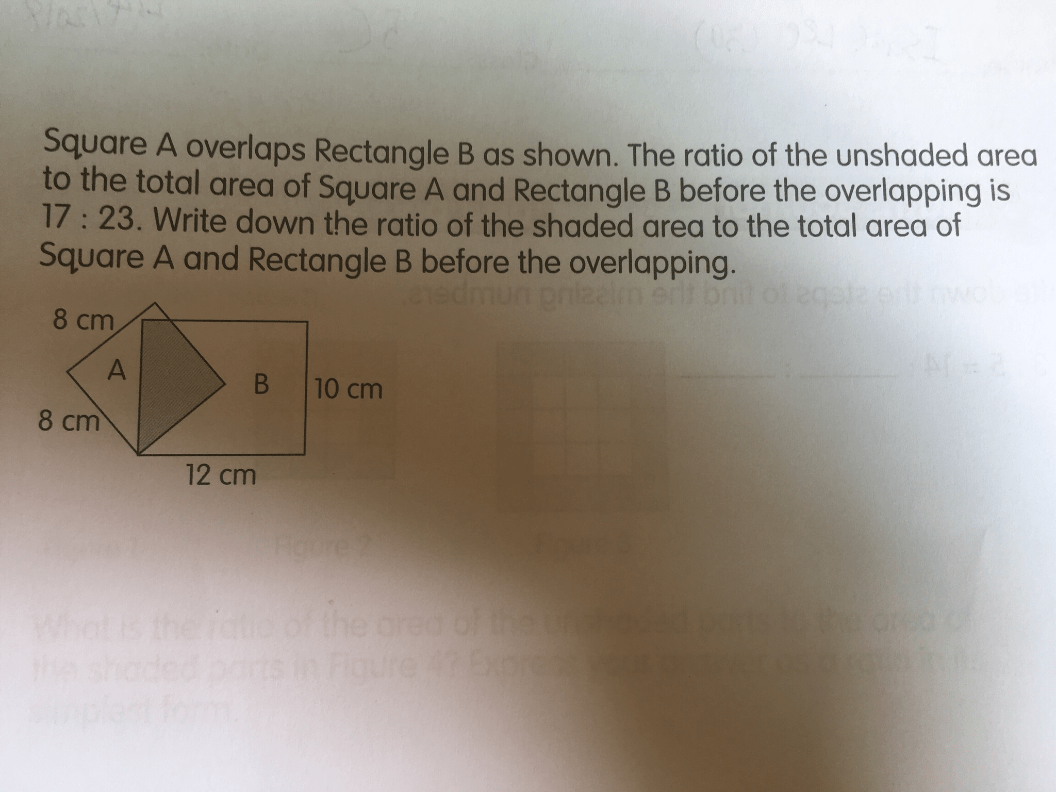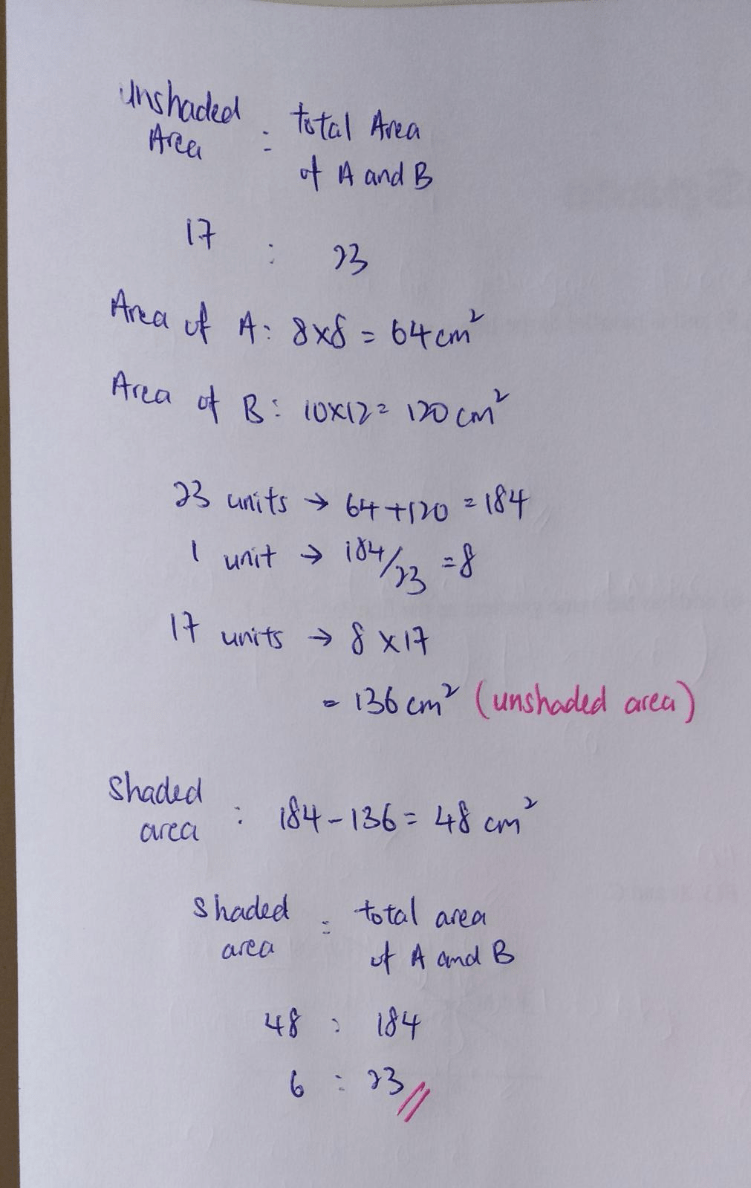# QuestionHelp needed. Tks

= 23 : 17 :  23-17 :       6/2 : 3 : 3

= 2317 : 6 :       3 : 3 : 3

Actually I disagree with all the answers above.

• The question states that “the ratio of the unshaded area to the total area of square A and rectangle B before the overlapping is 17:23″.
• It then asks the “the ratio of the shaded area to the total area of square A and rectangle B before the overlapping“.

Both the above are “before the overlapping”.

So if the unshaded area is 17 parts before the overlapping, then the shaded area must be 6 parts, if there are 23 parts in total.

The argument that the shaded area is 3 parts only works if the first part of the question is reworded to “the ratio of the unshaded area to the total area of square A and rectangle B after the overlapping is 17:23″.

0 Replies 0 Likes

unshaded area : total area of A and B
17 : 23

This means,
total area of A and B = 23 units

Therefore,
total area of A and B – unshaded area will give you 2 times of the shaded area. (this is because the shaded area is currently being overlapped by part of A and part of B)
23 units – 17 units = 6 units

We now only need to find 1 times of shaded area, so 1 times of shaded area = 6/2 = 3 units

Therefore, the ratio of shaded area : total area of A and B is 3 : 23

0 Replies 0 Likes

Ans : 3 : 23.

Note :

23 – 17 = 6 (shaded area of Square A + shaded area of Rectangle B)

6/2 = 3 (overlapping shaded area as shown in the figure)

shaded area : total area of Square A and Rectangle B before the overlapping

3 : 23

0 Replies 0 Likes